# GSEB Solutions Class 10 Maths Chapter 13 Surface Areas and Volumes Ex 13.2

Gujarat Board GSEB Solutions Class 10 Maths Chapter 13 Surface Areas and Volumes Ex 13.2 Textbook Questions and Answers.

## Gujarat Board Textbook Solutions Class 10 Maths Chapter 13 Surface Areas and Volumes Ex 13.2

Question 1.
A solid is in the shape of a cone standing on a hemisphere with both their radii being equal to 1 cm and the height of the cone is equal to its radius. Find the volume of the solid in terms of it.
Solution:
h = r
r = 1 cm
h = 1 cm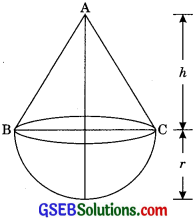Volume of solid
= Volume of cone + Volume of hemisphere
= $$\frac {1}{3}$$πr2h + $$\frac {2}{3}$$πr3
= $$\frac {1}{3}$$π x 12 x 1 + $$\frac {2}{3}$$π = $$\frac {π + 2π}{3}$$
= $$\frac {3π}{3}$$ = π cm3

Question 2.
Rachel, an engineering student, was asked ta make a model shaped like a cylinder with two cones attached at its two ends by using a thin aluminum sheet. The diameter of the model is 3 cm and its length is 12 cm. If each cone has a height of 2 cm, find the volume of air contained in the model that Rachel made. (Assume the outer and inner dimension of the model to be nearly the same).
Solution:
Diameter of cylindrical model = 3 cm
r = $$\frac {3}{2}$$cm
Height of cylindrical part of the model
= 12 – (2 + 2)
h1 = 8 cm
h2 (height of cone) = 2 cm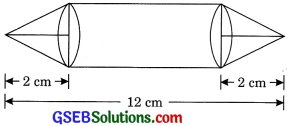and radius r = $$\frac {3}{2}$$ cm (radius of both parts is same)
Volume of air contained in the model
= Volume of cylindrical part + Volume of two conical parts
= πr2h1 + 2 x $$\frac {1}{3}$$r2h2
= π($$\frac {3}{2}$$)2 x 8 + $$\frac {2}{3}$$π x ($$\frac {3}{2}$$)2 x 2
= π x $$\frac {9}{4}$$ x 8 + $$\frac {2}{3}$$π x $$\frac {9}{4}$$ x 2
= 18π + 3π = 21π
= 21 x $$\frac {22}{7}$$ = 3 x 22 = 66 cm3Question 3.
A gulab jamun, contains sugar syrup up to about 30% of its volume. Find approximately how much syrup would be found in 45 gulab jamuns, each shaped like a cylinder with two hemispherical ends with length 5 cm and diameter 2.8 cm.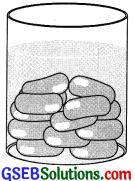Solution:
Total length of gulab jamun = 5 cm
Diameter = 2.8 cm
r = 1.4 cm
Height of cylindrical portion of gulab jamun
= 5 – (1.4 + 1.4)
= 5 – 2.8 = 2.2 cm
Volume of one gulab jamun = Volume of cylindrical portion + Volume of two hemispherical ends
πr2h + 2 x $$\frac {2}{3}$$πr3
= $$\frac {22}{7}$$ x (1.4)2 x 2.2 + $$\frac {4}{3}$$ x $$\frac {22}{7}$$ x (1.4)3
= $$\frac {22}{7}$$ x (1.4) x 1.4 + 2.2 + $$\frac {4}{3}$$ x $$\frac {22}{7}$$ x 1.4 x 1.4 x 1.4
= 22 x 0.2 x 1.4 x 2.2 + $$\frac {4}{3}$$ x 22 x 0.2 x 1.4 x 1.4
= 13.552 + 11.498
= 25.05 cm3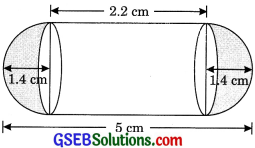Volume of 45 gulabjamuns
= 45 x 25.05
= 1127.25
Volume of sugar syrup in 45 gulab jamuns
= 30% of 1127.25
= $$\frac {22}{7}$$ x 1127.25 = 338.175
= 338.2 cm3 (aprox.)Question 4.
A pen stand made of wood is in the shape of a cuboid with four conical depression to hold pens. The dimensions of the cuboid are 15 cm by 10 cm by 3.5 cm. The radius of each of the depression is 0.5 cm and the depth is 1.4 cm. Find the volume of wood in the entire stand.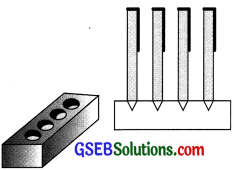Solution:
Length of pen stand l = 15 cm
and height h = 3.5 cm
Volume of cuboidal wood = l x b x h
= 15 x 10 x 3.5 = 525 cm3
Radius of conical depression r = 0.5 cm
and height h = 1.4 cm
Volume of one conical depression
$$\frac {1}{3}$$ πr2h
Volume of four conical depressions
= 4 x $$\frac {1}{3}$$πr2h
= $$\frac {4}{3}$$ x $$\frac {22}{7}$$ x (0.5)2 x 1.4
= $$\frac {88}{3}$$ x 0.5 x 0.5 x 0.2
= $$\frac {4.4}{3}$$ cm3 = 1.47 cm3
Volume of wooden pen stand
= Volume of wood – Volume of conical depressions
= 525 – 1.47
= 523.53 cm3Question 5.
A vessel is in the form of an inverted cone. Its height is 8 cm and the radius of its top, which is open is 5 cm. It is filled with water up to the brim. When lead shots, each of which is a sphere of radius 0.5 cm are dropped into the vessel, one-fourth of the water flows out. Find the number of lead shots dropped in the vessel.
Solution:
For cone, h = 8 cm
r = 5 cm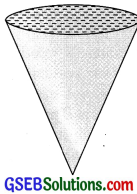Volume of water filled in the cone up to the brim = $$\frac {1}{3}$$πr2h = $$\frac {1}{3}$$π x 52 x 8
Volume of 1 lead shot = $$\frac {4}{3}$$πr3 = $$\frac {4}{3}$$π x (0.5)3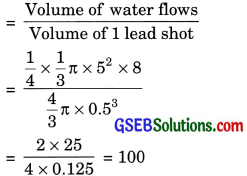Question 6.
A solid iron pole consists of a cylinder of height 220 cm and base diameter 24 cm, which is surmounted by another cylinder height 60 cm and radius 8 cm. Find the mass of the pole, given that 1 cm3 of iron has approximately 8 g mass. (Use ir=3.14)
Solution:
Diameter of cylinder A = 24 cm
So radius r1 = 12 cm
And height h1 = 220cm
Radius of cylinder B = 8 cm
i.e., r2 = 8 cm
and height h2 = 60 cm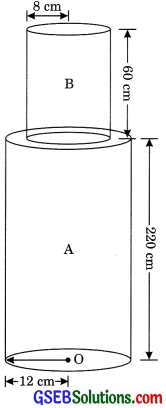Volume of pole
= Volume of cylinder A + Volume of cylinder B
= πr12h1 + πr12h2
= π [r12h1 + r12h2]
= 3.14 [122 x 220 + 82 x 60]
= 3.14 [144 x 220 + 64 x 60]
= 3.14 x 35520
= 11532.8 cm3
Mass of pole = Volume x Density
= 11532.8 x 8
= $$\frac{11532.8 \times 8}{1000}$$
= 892.26 kgQuestion 7.
A solid consisting of a right circular cone of height 120 cm and radius 60 cm standing on a hemisphere of radius 60 cm is placed upright in a right circular cylinder full of water such ‘ that it touches the bottom. Find the volume of water left in the cylinder, if the radius of the cylinder is 60 cm and its height is 180 cm.
Solution:
Height of cone h = 120 cm
Radius of hemisphere = 60 cmVolume of solid = Volume of cone + Volume of hemisphere
= $$\frac {1}{3}$$ πr2h + $$\frac {2}{3}$$πr3 = $$\frac {1}{3}$$πr2 (h + 2r)
= $$\frac {1}{3}$$ x 3.14 x 60 x 60 x (120 x 2 x 60)
= 3.14 x 60 x 60 x (120 + 2 x 60)
= 3.14 x 20 x 60 x (120 + 120)
= 904320 cm3
Volume of cylindrical vessel = πr2h
= 3.14 x 602 x 180
= 3.14 x 60 x 60 x 180 = 2034720 cm3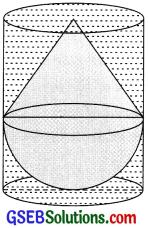Volume of water left
= 2034720 – 904320 = 1130400 cm3
= $$\frac{1130400}{100 \times 100 \times 100}$$m3 = 11304 m3

Question 8.
Aspherical glass vessel has a cylindrical neck 8 cm long, 2 cm in diameter, the diameter of the spherical part is 8.5 cm. By measuring the amount of water it holds, a child finds its volume to be 345 cm3. Check whether she is correct, taking the above as the inside measurements, and π = 3.14.
Solution:
Cylindrical neck of vessel has diameter = 2 cm
r = 1 cm
h = 8 cm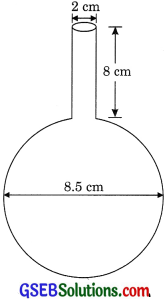Volume of cylindrical portion = πr2h
= 3.14 x 12 x 8 = 25.12 cm3
Spherical part has diameter = 8.5 cm
r = $$\frac {8.5}{2}$$ = 4.25 cm
Volume of spherical part = $$\frac {4}{3}$$ πr3
= $$\frac {4}{3}$$ x 3.14 x (4.25)3
= $$\frac{964.176}{3}$$ = 321.392 cm3
Total volume of vessel
= 25.12 + 321.392
= 346.51 cm3
Not correct. The correct answer is 346.51 cm3.